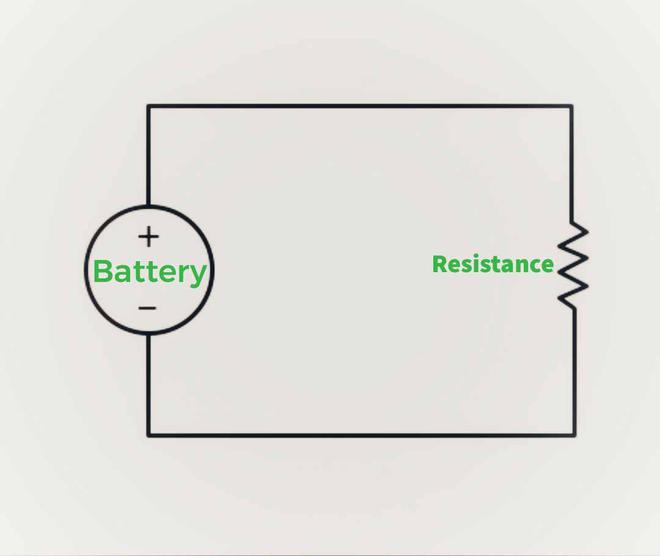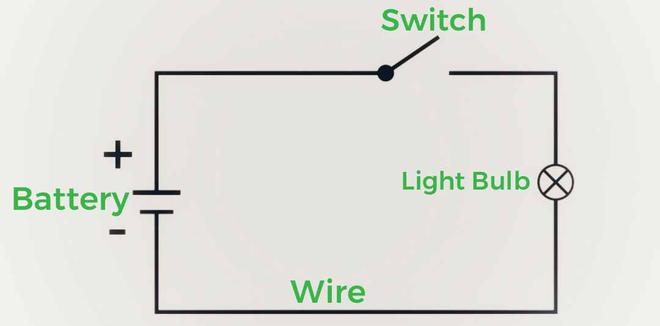Open in App
Not now

## Related Articles

• CBSE Class 10 Syllabus (All Subjects)
• CBSE Class 10 Maths Notes
• CBSE Class 10 Science Revision Notes
• CBSE Class 10 Social Science Revision Notes

# Electric Circuit – Definition, Components, Circuit with Bulbs

• Last Updated : 09 Nov, 2021

When the matter is held in an electric or magnetic field, it has an electric charge, which causes it to experience a force. An electric charge has an electric field associated with it, and a moving electric charge produces a magnetic field. The electromagnetic field is a combination of electric and magnetic fields. The electromagnetic force, which is the cornerstone of physics, is created by the interaction of the charges.

Electric Current: The rate of flow of electric charges is used to express electric current. The quantity of charge moving across a specific area in a given amount of time is referred to as the rate of flow.

If at time t, a net electric charge (Q) passes across a conductor’s cross-section, then Electric Current(I) is represented as:-

I = Q/t

Where I represents electric current, Q represents net charge, and t represents time in seconds. The ampere is the SI unit of current. (Defined as the flow of 1 Coulomb of charge per second)

Electric potential: It is the amount of effort required to move a positive charge from one location to another. A multimeter is electronic measuring equipment that measures electric potential difference, electric current, and electric resistance, among other things.

Ohm’s Law: If all physical parameters and temperature stay constant, Ohm’s law asserts that the voltage across a conductor is directly proportional to the current flowing through it. Mathematically this relation is written as,

V = IR

The Junction Law: It states that the sum of currents entering and leaving the junction must match the sum of currents leaving it.

The Loop Law: It states that in a closed circuit loop, the algebraic total of the potential differences across multiple components must be zero.

### Electric Circuit

Alessandro Volta invented the first electric circuit in 1800. Volta discovered that by connecting bowls of salt solution with metal strips, he could create a continuous flow of electricity. Later, he made his voltaic pile out of alternating discs of copper, zinc, and cardboard that had been soaked in a salt solution (an early battery). By connecting a wire from top to bottom, he was able to make the electric current flow across the circuit. Electrolysis was the first practical application of electric current, which led to the discovery of new chemical elements.

An electric circuit is a closed-loop or channel that connects electrical components and allows electrons to flow.

An electric circuit is a collection of conducting elements that are designed to carry electric current for a certain purpose. This route is constructed with electrical cables and is powered by a battery. The source is the point where electrons begin to flow, and the return is the point where electrons depart the electrical circuit.

### Components of Electric Circuit

The power source, conductors, switch, and load make up a basic circuit.Simple Electric Circuit

1. Battery/Cell – It is the power source of the circuit.
2. Load – Resistance(eg Light Bulb)
3. Conductors – They’re made of copper wires that haven’t been insulated. The load is connected to the power source on one end of the cable, and the power source is connected to the load on the other.
4. Switch –  A switch is a device that allows you to open or close a circuit.

### Electric Circuit with Bulb

To make an Electric Circuit with Bulb following things are required:-

• Electric Bulb
• Wire
• Cell/Battery
• Key/SwitchElectric Circuit with a bulb

Connect the wires to the bulb and one end of the wire to the battery; observe what occurs; the bulb will not change; however, when you connect the free wire to the battery and complete the circuit, the bulb will begin to glow; so, the circuit must be completed in order for the current to flow. Only when there is at least one closed loop from the positive to the negative end of an electrical circuit is it considered complete. This is the most basic type of electric circuit; the circuit inside a television is more intricate and has more components.

Electric current flows only when there is a continuous connection from a battery to various components and back to the battery, any disruption will cause the current to cease flowing.

Electric batteries should also be handled with caution; never connect the battery’s two terminals without a bulb or a load, as the chemicals inside the battery react so quickly that they generate a tremendous amount of energy, which can cause the battery to explode.

### Sample Questions

Question 1: What are Greek words from which the word ” Electricity ” is derived?

Electricity was described using the Greek words “Electrica” and “Elektron.”

Question 2: What is electric current?

The rate of flow of electric charges is used to express electric current. The quantity of charge moving across a specific area in a given amount of time is referred to as the rate of flow.

I = Q/t

Where I represents electric current, Q represents net charge, and t represents time in seconds.

Question 3: Define Electric Potential.

Electric potential is the amount of effort required to move a positive charge from one location to another.

Question 4: Who invented the first electric circuit?